# Class 8 NCERT Solutions – Chapter 15 Introduction to Graphs – Exercise 15.2

### Question 1: Plot the following points on a graph sheet. Verify if they lie on a line

(a) A(4, 0), B(4, 2), C(4, 6), D(4, 2.5)

Solution: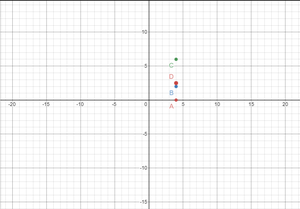The points A(4, 0), B(4, 2), C(4, 6), D(4, 2.5) lie on a line and the line is parallel to Y-axis.

(b) P(1, 1), Q(2, 2), R(3, 3), S(4, 4)

Solution: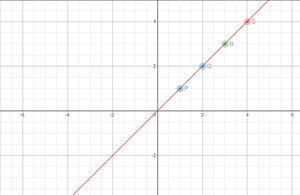The points P(1, 1), Q(2, 2), R(3, 3), S(4, 4) lie on a line and the line is passing through origin.

(c) K(2, 3), L(5, 3), M(5, 5), N(2, 5)

Solution: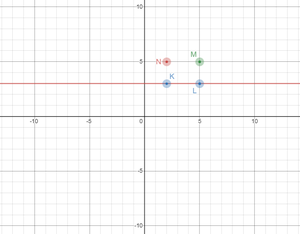The points K(2, 3), L(5, 3), M(5, 5), N(2, 5) do not lie on a line.

### Question 2: Draw the line passing through (2, 3) and (3, 2). Find the coordinates of the points at which this line meets the x-axis and y-axis.

Solution: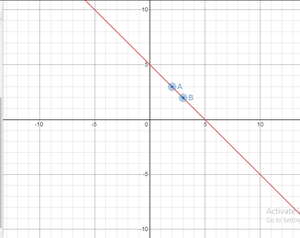The line meets x-axis at (5, 0) and the line meets y-axis at (0, 5).

### Question 3: Write the coordinates of the vertices of each of these adjoining figures.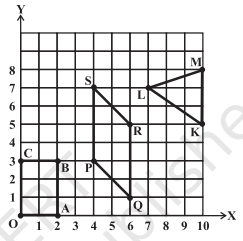Coordinates of the vertices of the figure PQRS: P(4, 3), Q(6, 1), R(6, 5), S(4, 7).

Coordinates of the vertices of the figure OABC: O(0, 0), A(2, 0), B(2, 3), C(0, 3).

Coordinates of the vertices of the figure KLM: K(10, 5), L(7, 7), M(10, 8).

### Question 4: State whether True or False. Correct that are false.

(i) A point whose x coordinate is zero and y-coordinate is non-zero will lie on the y-axis.